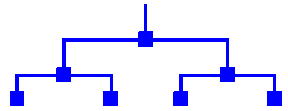# binary tree

Also found in: Acronyms, Wikipedia.

## binary tree

[′bīn·ə·rē ′trē]
(mathematics)
A rooted tree in which each vertex has a maximum of two successors.
McGraw-Hill Dictionary of Scientific & Technical Terms, 6E, Copyright © 2003 by The McGraw-Hill Companies, Inc.

## binary tree

(btree) A tree in which each node has at most two successors or child nodes. In Haskell this could be represented as

data BTree a = NilTree | Node a (BTree a) (BTree a)

## binary tree

A data structure in which each node contains one parent and no more than two children. See quad tree and splay tree.

Binary TreeCopyright © 1981-2019 by The Computer Language Company Inc. All Rights reserved. THIS DEFINITION IS FOR PERSONAL USE ONLY. All other reproduction is strictly prohibited without permission from the publisher.
References in periodicals archive ?
The binary tree method is used to analyze the future cash flow of credit bonds, as shown in Figure 2.
Let T be the vid binary tree, [P.sub.r] be the spinning possibility of node r, S be the subtree spinning rate, and [T.sub.r] denote a subtree of T whose root node is r; we propose a heuristic spinning strategy as shown in Algorithm 2.
For example, when n=3, firstly forming two binary tree such as [Y.sub.2|0], generating 1 tree such as [Y.sub.1|1], lastly generating two trees such as [Y.sub.0|2].
Corollary 1 For any two pairs of twin binary trees with n leaves, there is a sequence of twin binary trees ([t.sub.1],[t'.sub.1]),..., ([t.sub.k], [t'.sub.k]) of size k = O(n) that starts with the first pair, ends with the second pair, and for every i (1 [less than or equal to] i < k), either [t.sub.i] = [t.sub.i+1] or [t.sub.i+1] is obtained from [t.sub.i] by a single tree-rotate operation (the same for [t'.sub.j] and [t'.sub.i+1]).
Binary Tree gives organizations a direct and predictable path to a successful technology transformation.
Create a binary tree T with the same spine structure as T([tau]) as follows.
Let (X, d) be a metric space with bounded geometry with an injective and Lipschitz map from the infinite binary tree T to X.
"We look forward to demonstrating our continued commitment to HP clients looking to migrate to the cloud," states Stefan af Bjur, Binary Tree General Manager, EMEA.
Because the judgment of MC directly affects the coding efficiency for B-frame prediction, we combine this method with MI to detect scene cuts; then, the GOP is adaptively and proportionately set by the analysis of MC in a scene, and the temporal scalability of a flexible sized GOP is achieved by a proposed binary tree algorithm.
Basically, a CART is a binary tree that uses a set of yes/no questions to construct its nodes by splitting an observation into two parts that are as homogenous as possible and then repeating the process for each resulting part until complete decomposition of the observation is achieved.
We explore AHC to the RSSI and LQI to generate a binary tree (also named "Dendrogram" ) derived from the architecture of the data, hoping to directly observe the number of clusters by counting the number of branches of this tree.

Site: Follow: Share:
Open / Close